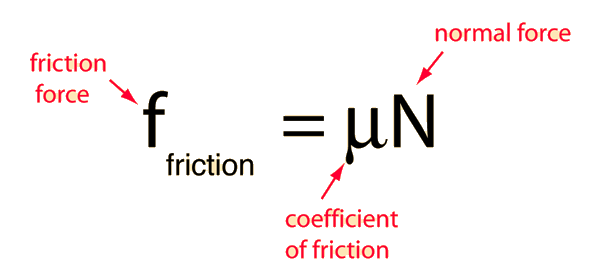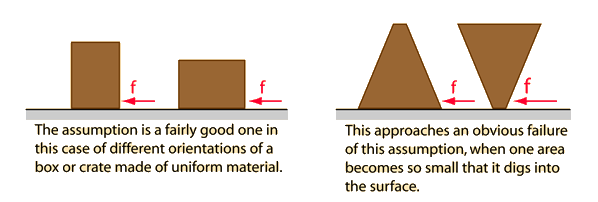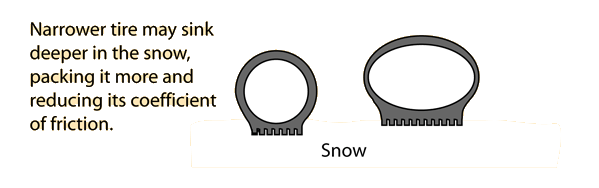# Friction Assumptions

In the standard model for friction between surfaces, there are certain inherent assumptions:1. The frictional force is independent of area of contact Exceptions 2. The frictional force is independent of the velocity of motion Exceptions 3. The frictional force is proportional to the normal force. Exceptions

One can readily find circumstances where any or all of these assumptions are invalid, but they provide a straightforward framework for a beginning treatment of friction that satisfactorily describes many phenomena.

Index

Friction concepts

 HyperPhysics***** Mechanics R Nave
Go Back

# Friction and Area of Contact

Part of the standard model of surface friction is the assumption that the frictional resistance force between two surfaces is independent of the area of contact.While exceptions exist, the assumption has enough validity to be useful in many circumstances. For example, it implies that wide tires will not in general give better traction than narrow tires, and will not change the normal braking distance for a car. Better traction can be obtained with wide tires, or tires with lower pressure, if the pressure changes the coefficient of friction, as on a surface of snow.

Index

Friction concepts

 HyperPhysics***** Mechanics R Nave
Go Back

# Friction and Velocity

Part of the standard model of surface friction is the assumption that the frictional resistance force between two surfaces is independent of the velocity of relative movement. While this is approximately true for a wide range of low speeds, as the speed increases and air friction is encountered, it is found that the friction not only depends on the speed, but upon the square and sometimes higher powers of the speed. If there are fluid lubricants involved, then viscous resistance is encountered, and it is speed dependent.

Like the other standard assumptions, exceptions are easy to find, but the assumption is nevertheless useful for modeling a wide variety of circumstances where two solid materials move relative to each other.

Index

Friction concepts

 HyperPhysics***** Mechanics R Nave
Go Back

# Friction and Normal Force

Part of the standard model of surface friction is the assumption that the frictional resistance force between two surfaces is proportional to the normal force pressing them together. A common exception occurs in snow where greater traction occurs by having wider tires with lower pressure. The same normal force is exerted, but the traction (friction) is greater with the wider tire since it doesn't pack the snow as much. The packing of the snow tends to depend on the pressure exerted on the snow, and supporting the same weight on a smaller area implies that more pressure is exerted. This example is also an exception to the assumption of independence of area of contact. With a given area of contact, increasing the normal force will pack the snow, decreasing the effective coefficient of friction.While exceptions such as this are easily found, the assumption that friction is proportional to the normal force is still reasonably valid in many cases and forms a useful model for many circumstances.

A valid question is why does the frictional resistance increase proportionally with normal force? Jones and Childers have a thoughtful discussion of this. They point out that the area of coverage for a sliding block is actually much larger than the area of contact. Because of microscopic irregularities in the surface, the block is actually riding on a small fraction of the coverage area. Because such points of contact are deformable, an increase in normal force will actually increase the area of actual contact and increase the resistance to movement.

Index

Friction concepts

Reference
Jones & Childers, 2nd Ed.
Sec 4.8

 HyperPhysics***** Mechanics R Nave
Go Back